With the rising popularity of bitcoin, more and more analysts are trying to develop a better understanding of this phenomenon. While it might be very difficult to make accurate predictions of the actual bitcoin prices, it is still possible to identify some interesting trends and relationships. In what follows, I will demonstrate how to use the Minitab Predictive Analytics Module to accomplish this task.

### Learn about the Minitab Predictive Analytics Module in our on-demand webinar: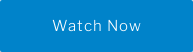hbspt.cta._relativeUrls=true;hbspt.cta.load(3447555, 'c434fb3a-b055-4d94-b1a9-de272e801a27', {"useNewLoader":"true","region":"na1"});

The actual bitcoin data is available from many public sources. One very useful dataset can be downloaded here

The dataset includes bitcoin statistics on daily basis going all the way back to 2009. Each day is summarized by 44 different metrics, including bitcoin price, various fees, block count, transaction count, return on investment, and more.

For the purposes of our analysis, I will look at the bitcoin daily statistics from January 1, 2015 to April 20, 2021. This eliminates some of the earlier history which could detract from the most recent trends. The dataset includes a variable called ROI30d – a percent return on investment for the asset assuming a purchase 30 days prior. In what follows, my main objective will be to make accurate predictions of the 30-day return on investment using the remaining variables as potential predictors.

## Data Summaries

First, let me have a quick look at various data summaries using Minitab.

Below is the time series plot of the 30-day return on investment: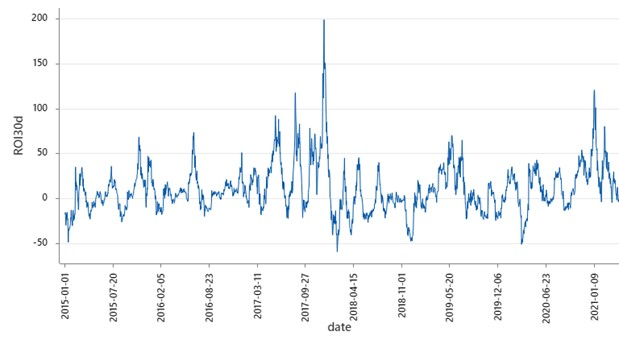As you can see, investing in Bitcoin can provide lucrative returns or significant losses. Given the volatility of this asset, timing of an investment in Bitcoin is critical to the return. So knowing what impacts the return can help determine when would be the best time to invest.

## Determining The Most Important Predictors

So often we are asked questions and need to come up with the best answer in the shortest amount of time. With 44 possible predictors, I need to know which ones matter the most, and I need to know it quickly so I can run an analysis.

That’s exactly why the Minitab Predictive Analytics Module has an option called “Discover Key Predictors.” This option allows me to let the software identify the most important variables, enabling me to build a model that is still highly accurate and yet far less complex, making it much more user friendly.

I take my data set and run it through the TreeNet "Discover Key Predictors." As expected, Minitab starts with the supplied set of candidate predictors and proceeds by building a series of models in sequence, and each subsequent model uses one less predictor by dropping the least important variable. Thus, the entire process is a modern generalization of the backwards elimination process known from classical regression modeling. Here is what happens when I start with the complete set of predictors (excluding date):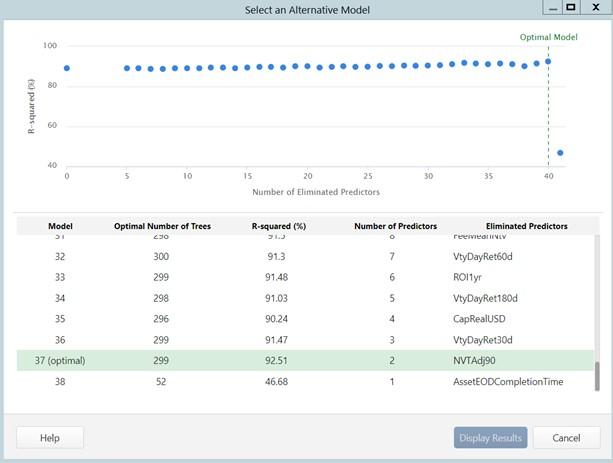Looking at the graphical visualization of my possible models, you can see that the model accuracy fluctuates around 90% until only two predictors are left. When I dig into the analysis, the "Discover Key Predictors" reveals that AssetEODCompletionTime is the “last man standing” or the most important predictor.

Unfortunately, looking up its definition from the data dictionary, this “predictor” is simply the time the last data was collected each day, which isn’t a helpful metric. As a result, I’d like to eliminate this because I know – with certainty – that these are likely correlated but not predictive. This is not uncommon in predictor selection: often times the machine first selects a bunch of useless players. This example also highlights the importance of pairing predictive analytics with subject matter expertise. Fortunately, the solution is simple – just drop it from the starting list of variables and redo the “Discover Best Predictor” analysis!

After dropping AssetEODCompletionTime from the original list and restarting the predictor discovery process, I obtain the following summary: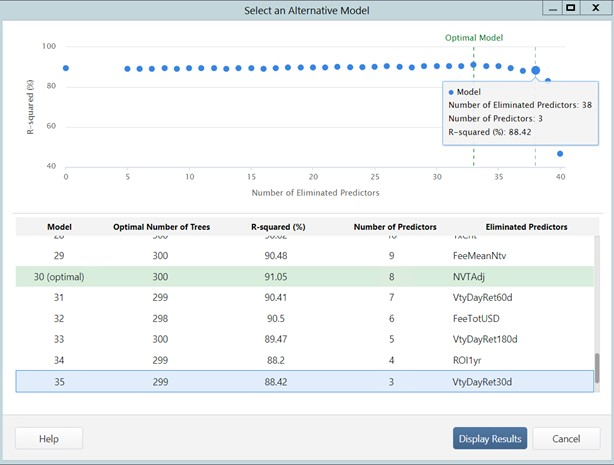Note that Minitab’s engine highlights that the optimal model uses 8 original variables (metrics) and achieves 91% R-squared on the 50% test partition. This is an excellent performance result for a regression model of this type! Also note that there is a statistical variation in the model performances around 90%.

Minitab also gives me a helpful visualization that the overall accuracy of models only drops significantly when the number of predictors falls below 3. For the sake of building the simplest model, while maximizing accuracy, I select a model with 3 predictors for more detailed analysis.   Alternatively, you can remove some of these variables from the original candidate list and redo the best predictor search to identify a different subset of winners. Remember, in this example I’m trying to identify what matters quickly. If maximum accuracy is your objective, you would probably go with the optimal model, instead. The opportunities are endless, and no matter what your objective is, you can accomplish it easily with only a few clicks!

## Modeling Insights

Back to my example. I will now take a closer look at the 3-variable model selected above. Here is the summary performance of this model: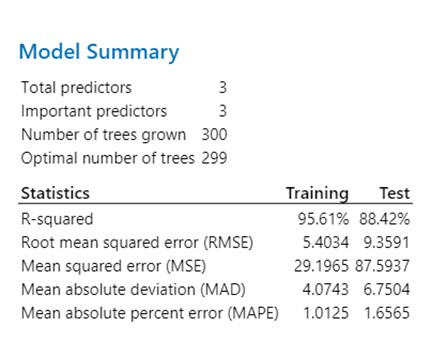As you can see, we have 88%+ accuracy on the 50% test sample – an excellent result! Furthermore, Minitab reports relative rankings of the three surviving metrics in terms of their overall contribution into this model: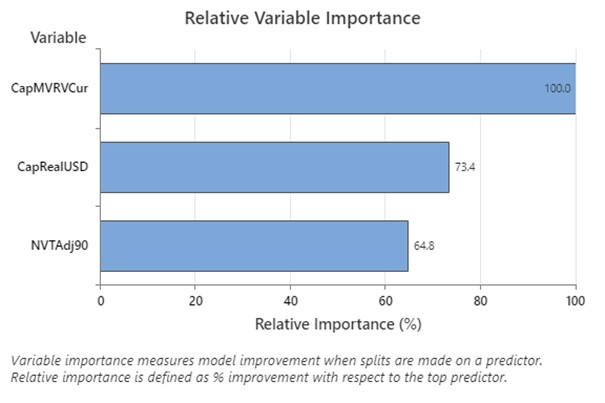The topmost important variable associated with the 3-day return on investment is CapMVRVCur. It turns out that this variable summarizes possible overvaluation/undervaluation of the market. Here is the time series plot of this variable over the past 6 years: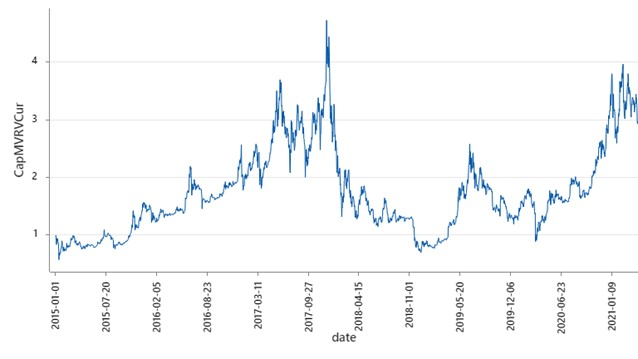It appears that this metric tends to fluctuate between 1.0 and 4.0 with the current values around 3.3 and possibly decreasing. Here is a more detailed description on this metric from the data dictionary:

The intuition behind the creation of this ratio was to divide a price function by a ‘fundamental’ as proxied by Realized Capitalization (see Capitalization, realized, USD). This gives you a ratio potentially indicating periods of overvaluation (when network value far exceeds its historical relationship to realized cap) and undervaluation. Realized cap is a potent fundamental as it can be understood as the average cost basis for holders at a given time, so the ratio of the two indicates whether holders are underwater or not, giving insight into aggregate sentiment.

TreeNet gradient boosting model also reveals the nature of contribution of this metric into the 30-day return on investment: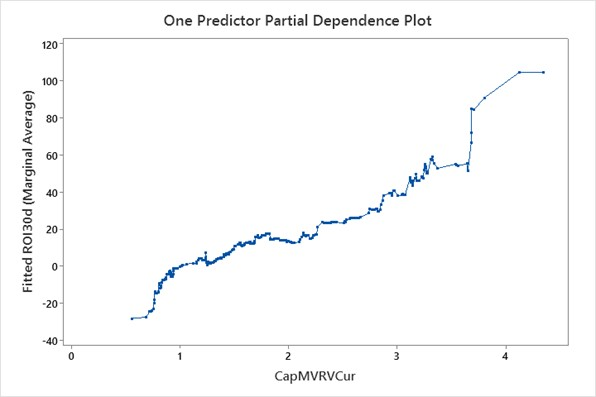Recall, that the most recent values of this metric are fluctuating around 3.3 and will possibly continue decreasing. From the above dependency plot, it is clear that if this indeed is going to be the case then we expect the 3-day ROI continue to decline. Alternatively, if there is any reason to believe that this metric is going to increase to 3.7 and above, we might expect a significant jump in the ROI, based on the historic pattern.

The above series of steps mimics a typical scenario encountered in predictive analytics. We started with a dataset containing 44 variables and quickly found the most important predictors in a matter of minutes. The Key Predictor Selection creates a short-cut to avoid the potentially tedious and laborious process of looking at each variable one at a time. Furthermore, TreeNet gradient boosting model showed superb accuracy. All of this highlights the power of modern predictive analytics and shows why you need it moving forward!